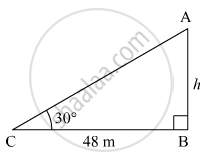Advertisement Remove all ads

# A Boy Standing at a Distance of 48 Meters from a Building Observes the Top of the Building and Makes an Angle of Elevation of 30°. Find the Height of the Building. - Geometry

Advertisement Remove all ads
Advertisement Remove all ads
Advertisement Remove all ads

A boy standing at a distance of 48 meters from a building observes the top of the building and makes an angle of elevation of 30°. Find the height of the building.

Advertisement Remove all ads

#### Solution

Let AB be the building and C be the position of the boy from the building.
Suppose the height of the building be h m.Here, BC = 48 m and ∠ACB = 30º.
In right ∆ABC,
$\tan30^\circ = \frac{AB}{BC}$
$\Rightarrow \frac{1}{\sqrt{3}} = \frac{h}{48}$
$\Rightarrow h = \frac{48}{\sqrt{3}} = 16\sqrt{3} m$
Thus, the height of the building is
$16\sqrt{3}$

Concept: Heights and Distances
Is there an error in this question or solution?

#### APPEARS IN

Balbharati Mathematics 2 Geometry 10th Standard SSC Maharashtra State Board
Chapter 6 Trigonometry
Problem Set 6 | Q 6 | Page 139

#### Video TutorialsVIEW ALL 

Advertisement Remove all ads
Share
Notifications

View all notifications

Forgot password?